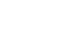# Calculate Earthquake Forces on Buildings and StructuresReading time: 1 minute

Earthquake resistant design requires calculation of earthquake forces on buildings and structures. The guide to earthquake resistant design of building and structures are given by IS 1893:2002 in India. In this article, how to calculate the earthquake forces for buildings and structures as per IS 1893:2002 code is discussed.## Calculate Earthquake Forces on Buildings and Structures

First step to calculate earthquake loads on structure is to identify the earthquake zone for which structure needs to be designed. This earthquake zones are displayed in a map on page – 6 of the code. After earthquake zone has been identified, the following steps are followed: 1. Calculate design horizontal seismic coefficient, Ah, which is given by (cl. 6.4.2 of IS1893 – 2002:Where, Z is the zone factor, given in table 2 of IS1893 – 2002. I is the importance factor of the structure depending on the function or use. This factor can be obtained from table 6 of the code. R is response reduction factor. This value is obtained from table 7 of the code. The value of 1/R shall not be more than one. Sa/g is average response acceleration coefficient. This value depends on time period of structure and on soil type. This can be obtained from clause 6.4.5 of the code. 2. Calculate design seismic base shear for the structure (VB). This is the total design lateral force along any principal direction. This is calculated as: VB = Ah x W Where Ah = horizontal seismic coefficient as calculated above in step 1. W = Total weight of the structure. 3. Now calculate the distribution of design forces on the structure. The seismic design base shear calculate in step above is distributed on the structure as design seismic forces. This is calculated as below:Where Qi = Design lateral force at floor i Wi = seismic weight of the floor i hi = height of the floor i from the base n = number of storeys of the building at which masses are located. 4. Distribution of horizontal seismic forces on structure: These forces are distributed on the vertical elements of the building resisting lateral forces.

### Example:

Consider a two-bay two-storied building for which earthquake forces need to be calculated. Consider total weight of the building as 1000 kN. Top roof has the weight of 200 kN and both floors have weight of 400 kN as shown in figure below. The calculation of earthquake seismic forces will be as shown below:Total Weight of the Structure Wi = 1000 kN Zone Factor (Zone IV) Z = 0.24 Importance factor (Table6) I = 1 Response Reduction Factor R = 3 Average response acceleration coefficient Let time period T 0.373358 (Cl. 7.6) Sa/g 2.5 Design Horizontal Seismic coefficient Ah 0.10 Base Shear 100 kN Storey Base Shear Height Wi Wixhi2 Qi Design Force 3 100 8.5 200 14450 57.00197 9.50 2 100 5 400 10000 39.44773 6.57 1 100 1.5 400 900 3.550296 0.59 Total 25350 (in kN)Read More: Soft Storey in Buildings and its Vulnerability Towards Earthquakes Performance of Various Types of Buildings during Earthquake Ductility of Building Structures for Earthquake Resistant Design Performance and Behavior of Masonry Structures during Earthquakes